# How to Subtract in ExcelClaudia Buckley#### Join the Excel conversation on Slack

Ask a question or join the conversation for all things Excel on our Slack channel.

Subtraction is one of the four basic arithmetic operations. It’s as easy to subtract values in Excel as it is in the real world - in some cases, easier, since Excel takes the mental aspect out of it with the use of formulas.

## The subtraction formula

A subtraction formula in Excel consists of the equal sign, the values or cell references to be used in the formula, and the minus sign, represented by a dash (-). The structure is:

``=number1 - number2``

A space may be placed between each element, but isn’t necessary. There is no SUBTRACT function in Excel.

You can subtract one number from another using explicit values,

``=1000-250``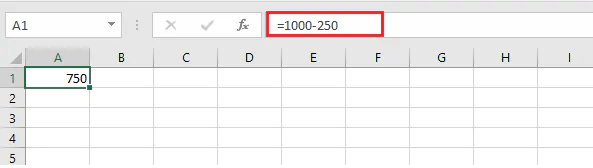or by making reference to the cells containing the values to be subtracted.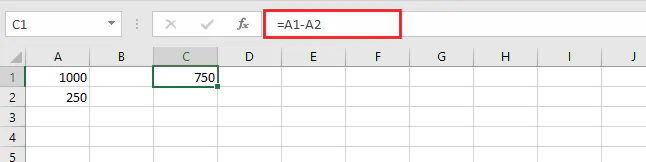As in the case of other formulas, if you choose to subtract numbers using cell references, you can simply click on the cell containing the relevant value where the formula calls for each number.

200+ Best Excel Shortcuts for PC and Mac

## Subtract two or more numbers

You may have a situation where you want to subtract two or more numbers, or numbers in a range. There is more than one way to do this.

• ### Subtract by listing numbers one at a time

In the example below, we can calculate net income by subtracting all the deductions from the gross income one by one, using the formula

``number1 - number2 - number3….``

Of course, we can use cell references as we learned before.

``=B1-B3-B4-B5-B6``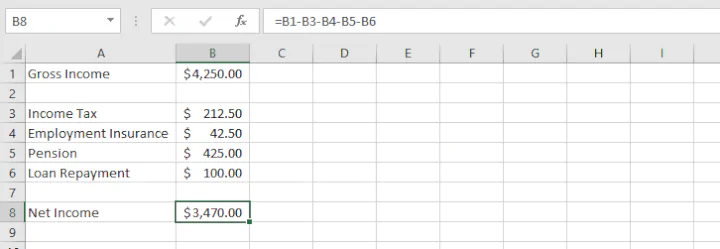• ### Subtract numbers as a range

The above method can get quite cumbersome if the list of numbers to be subtracted is a long one! We can treat the numbers in the list as a cell range instead, and use the SUM function to our advantage.

``=B1-SUM(B3:B6)``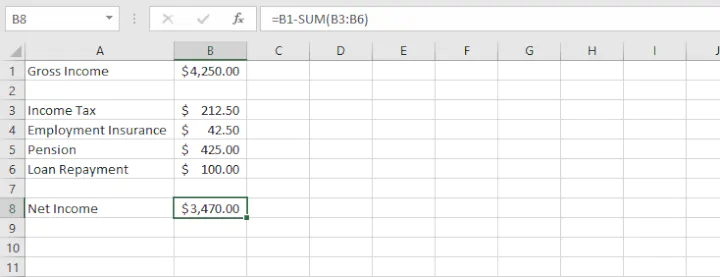Since the values to be deducted above are positive numbers, we’ve placed them in parentheses (so that this portion is calculated first). The SUM function adds the values within the B3 to B6 range. That total is then subtracted from the Gross Income in cell B1. The result is, of course, the same as using the longer method.

### Negative numbers

If the numbers you want to subtract are already listed as negatives, placing a minus sign before them converts them to positive (two negatives make a positive, right?).

To calculate Net Income where the deductions are already saved as negative numbers, placing a minus sign before them would result in a Net Income which is higher than Gross, which is of course, incorrect. The correct thing to do is to simply add all the numbers within the range, preferably by using the SUM function for the sake of efficiency.

``=SUM(B1, B3:B6)``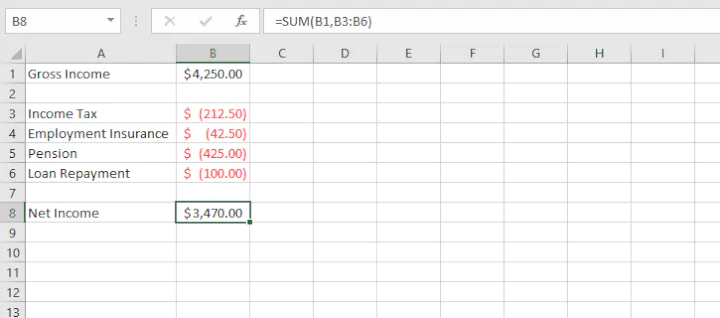## How to subtract numbers in columns

To subtract numbers in two columns row-by-row, write the standard subtraction formula in the output cell of the first row, then drag or double-click the fill handle to copy the formula to the remaining rows.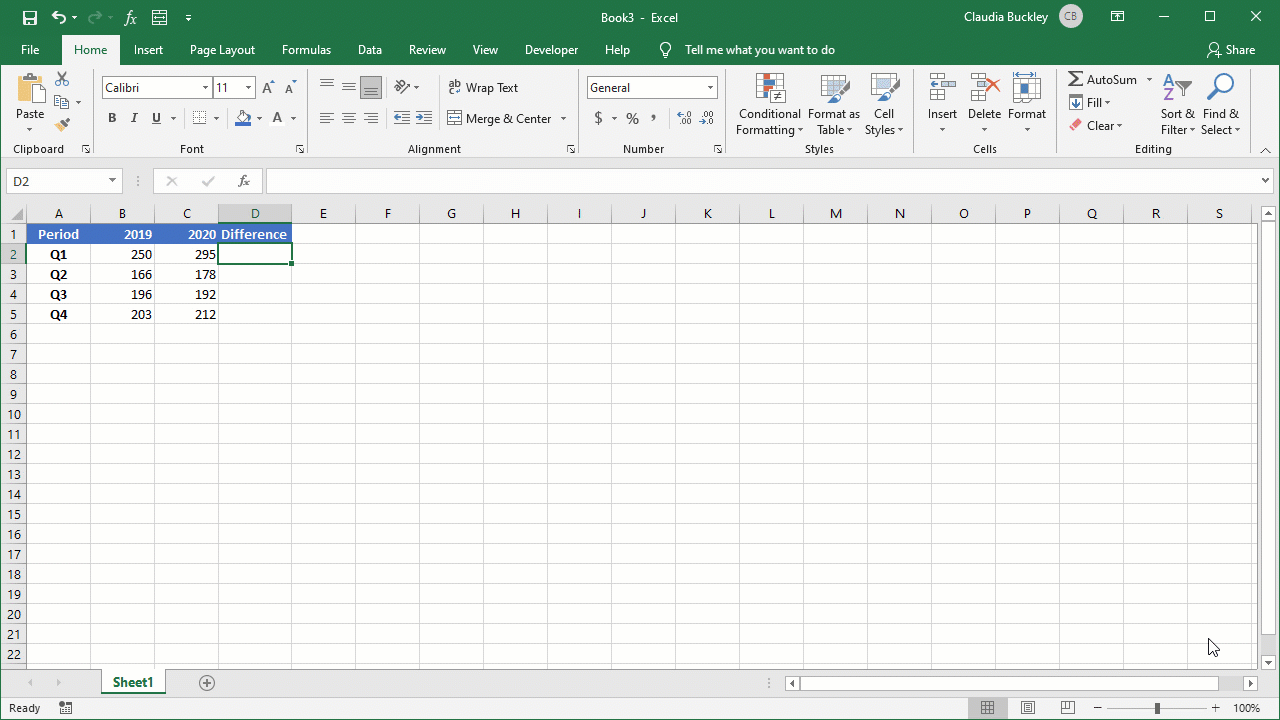Since the cell references are relative rather than absolute, the formula adjusts for each row so that the formula in cell D3 refers to the values C3 and B3, the formula in cell D4 refers to the values C4 and B4, and so on.

## How to subtract percentages

Subtracting percentages works in much the same way if we want to subtract, say, 30% from 90%. Just plug the numbers in using the formula above. But what if you want to decrease a number by a certain percentage?

Let’s say a retail store has a sale where certain products will be given a 10% discount off the original price. We could use the following formula:

``=original price * (1-discount%)``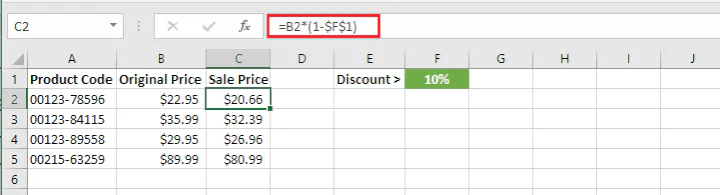In the example above, the reference to cell B2 is relative, but the reference to cell F1 is entered as an absolute reference since we do not want the formula to change to F2, F3, and so on when we copy downward.

## Subtract dates

Another common task is to subtract dates to find out how many days have elapsed (or will elapse) between one date and another. Excel can handle that too. Often, the simplest way is to just enter each date in a separate cell and subtract using the basic format we have been using.

At times, though, it may be advantageous to enter the dates themselves within the formula. This can be done using the DATE or DATEVALUE function so that Excel knows that the numbers it’s being asked to process are dates and should be treated as such.

For instance,

``=DATE(1965,3,9)-DATE(1963,4,30)``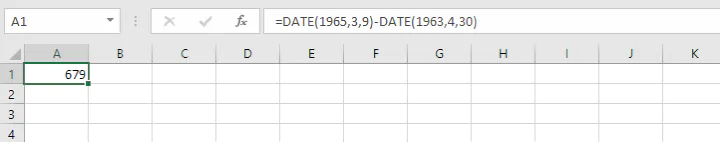The above formula calculates the number of days between March 9, 1965 and April 30, 1963. Note that the “larger” (more recent) date is placed before the “smaller” date to return a positive value, similar to subtracting integers.

An alternative to this method is

``=DATEVALUE("1965/3/9")-DATEVALUE("1963/4/30")``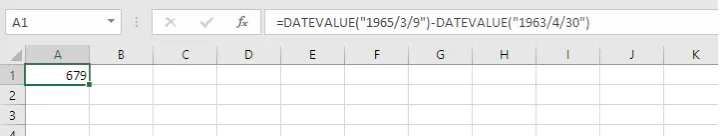Using the above formula asks Excel to treat a text string as a date and subtract one date from the other. Your computer’s regional setting will be used to determine whether months precede days or vice versa.

• Note: For calculations involving dates both before and after January 1, 1900 (e.g. calculate the number of days between July 4, 1776 and July 4, 2021), Excel will return an invalid result since January 1, 1900 is the first recognized date in Excel. If both dates are before January 1, 1900 or both dates are after January 1, 1900, it’s no problem.

## Subtract times

Subtracting times in Excel is similar to subtracting dates. The formula is:

``=end_time - start_time``

For example, to calculate the difference between the times in A1 and B1 below, where the time format has already been applied to both cells, use the formula:

``=B1-A1``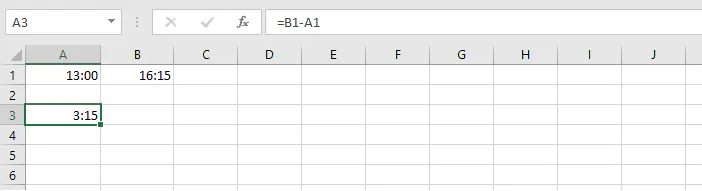Note that the later time is considered the greater value, so it is placed before the earlier time.

Alternatively, you can use the TIMEVALUE function and entering the time values directly into the formula as a text string.

``=TIMEVALUE("16:15")-TIMEVALUE("13:00")``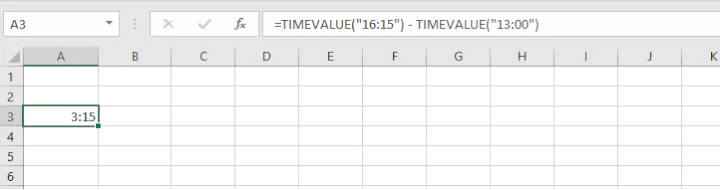or

``=TIMEVALUE("4:15 pm") - TIMEVALUE("1:00 pm")``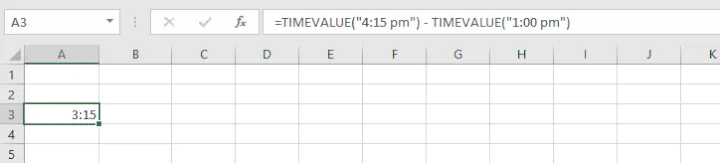Then make sure that the result cell is formatted to display the hours correctly by pressing Control 1 on your keyboard, and changing the Number Format to the Time category. Next, scroll through the available formats to select one which displays the number of hours, minutes and seconds elapsed then press OK.

## Subtract one matrix from another

For subtraction of matrices with the same number of rows and columns, the idea is to subtract corresponding values from each other, with the resulting matrix being of the same dimension. Below is an example: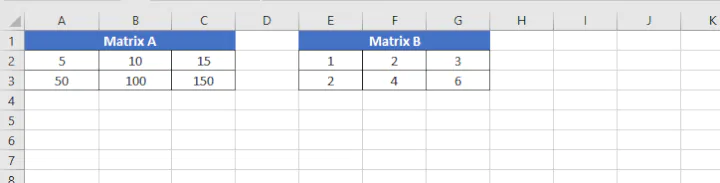Our aim here is to subtract E2 from A2, F2 from B2, and so on. We can accomplish this with a single array formula by doing the following:

1. Select a range of empty cells with the same dimensions as your matrices.
2. Type the matrix subtraction formula:
``=(A2:C3)-(E2:G3)``
1. Press Ctrl + Shift + Enter to make it an array formula.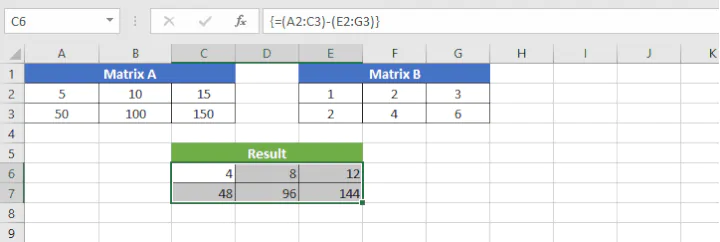Curly brackets are inserted around the formula by Excel, and the result is shown in the output range C6 to E7.

## Conclusion

Mastering the basic subtraction operation in Excel is one of the keys to simplifying complex tasks. Now that you’ve got this figured out, why not tackle your next challenge by leveling up on your other Excel skills? Check out our Basic and Advanced Excel course and other resources!

Become a certified Excel ninja with GoSkills bite-sized courses#### Join the Excel conversation on Slack

Ask a question or join the conversation for all things Excel on our Slack channel.Claudia Buckley

Claudia is a project manager and business skills instructor at GoSkills. In her spare time, she reads mystery novels and does genealogy research.